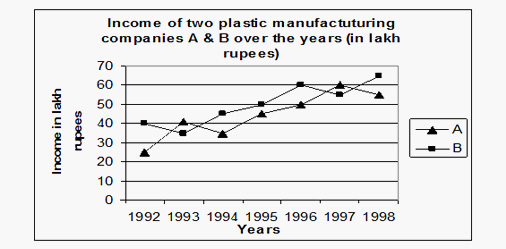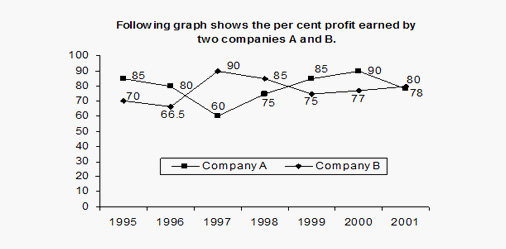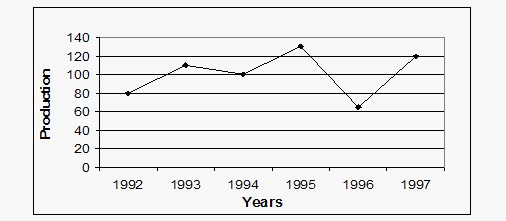# Line Graph Solved Examples

DIRECTIONS for questions 1-5: Study the following graph carefully and answer the questions given below (Profit is taken as the % of expenditure.)Example 1. What would be the ratio of income of company B in 1996 to the income of company A in 1993?
A. 9 : 10
B. 10 : 9
C. 3 : 2
D. 15 : 13
Example 2. If the expenditure of company B in 1997 is Rs. 50 lakhs, the percent profit earned by both the companies A and B in 1997 is equal, then what is the amount of profit earned by company A in 1997 (approximately)?
A. Rs. 5 lakhs
B. Rs. 4.5 lakhs
C. Rs. 5.5 lakhs
D. Rs. 6.2 lakhs
Example 3. If in the year 1995, company 'B' had a profit of 25%, what approximately was its expenditure in the year 1995 ?
A. Rs. 22 lakhs
B. Rs. 29 lakhs
C. Rs. 40 lakhs
D. Rs. 27 lakhs
Example 4. The average income of company 'B' per year is what percentage of the average income of company 'A' per year?(approximately)
A. 70%
B. 110%
C. 113%
D. 90%
Example 5. Income of company 'A' in 1996 is what percent of income of company 'B' in 1992?
A. 75%
B. 63.64%
C. 133.33%
D. 125%
DIRECTIONS for question 6-10: Study the following graph carefully and answer accordingly.Following graph shows the percent profit earned by two companies A and B on their investments.(Revenue = Investment + Profit)Example 6.Revenue of company B in 2000 was Rs.1239 lakhs. What was the investment in that year (in Rs lakhs) of company B?
A. 700
B. 800
C. 650
D. 193.03
Example 7. Investment of company B in 1998 was 20% more than that in the previous year. Profit in 1998 of company B was what per cent of its profit in 1997?
A. 10%
B. 102(2/3)%
C. 106(2/3)%
D. None of these
Example 8. In which of the following years is the ratio of investment and profit maximum for company A?
A. 2001
B. 1995
C. 1998
D. 2000
Example 9. If the revenue of company B in 1996 was same as the revenue of company B in 1999, what would be the ratio of investment of company B in 1999 to the investment of company B in 1996?
A. 9 : 10
B. 10 : 9
C. 13 : 15
D. 15 : 13
Example 10. In which of the following years was the investment minimum for company B?
A. 1995
B. 1999
C. 2000
D. Can't be determined
DIRECTIONS for questions 11 to 15: Given below are line graph examples with questions, which show the annual food grain production from 1992 to 1997. Refer to the graph & answer the questions based on line graph as given below.Example 11: What is an approximate percentage decrease in production from 1993 to 1994?
A. 87.5%
B. 37.5%
C. 9.09%
D. None of these
Example 12: The average production of 1994 and 1996 was approximately equal to production of which year?
A. 1996
B. 1992
C.1995
D. 1994
Example 13: The average production of the given years was more than to the production in how many years?
A. 1
B. 2
C. 3
D. 4
Example 14: If the production in 1992 is estimated to be 60% more than that in 1991, the estimate of 1991 production is?
A. 120 units
B. 100 units
C.60 units
D. 50 units
Example 15: The maximum increase in food grain production has been in
A. 1997
B. 1996
C.1995
D. 1994